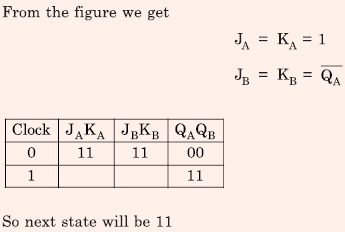# Digital Circuits - Online Test

Q1. Which of the following is an invalid state in an 8-4-2-1. Binary Coded Decimal counter
Explaination / Solution:

In binary coded decimal (BCD) counter the valid states are from 0 to 9 only in binary system 0000 to 1001 only. So, 1100 in decimal it is 12 which is invalid state in BCD counter.

Q2. A 10 kHz even-symmetric square wave is passed through a bandpass filter with centre frequency at 30 kHz and 3 dB passband of 6 kHz. The filter output is
Explaination / Solution:

10 KHz even symmetric square wave have frequency component present 10KHz, 30KHz, 50KHz, 70KHz [only odd harmonics due to half wave symmetry] Since bandpass filter is contered at 30KHz, 30KHz component will pass through filter output is nearly perfect cosine wave at 10 KHz Cosine in due to reason that signal in even signal.

Q3. The SOP (sum of products) form of a Boolean function is Σ(0,1,3,7,11), where inputs are A,B,C,D (A is MSB, and D is LSB). The equivalent minimized expression of the function is
Explaination / Solution: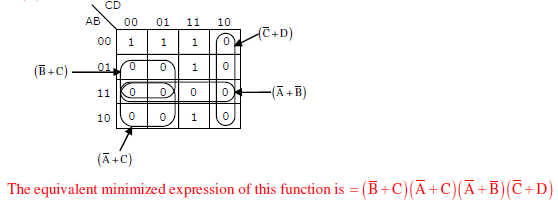Q4. A JK flip flop can be implemented by T flip-flops. Identify the correct implementation.
Explaination / Solution:Q5.
In an 8085 microprocessor, the following program is executed
2000H             XRA A
2001H             MVI B,04H
2003H             MVI A, 03H
2005H             RAR
2006H             DCR B
2007H             JNZ 2005
200AH             HLT
At the end of program, register A contains
Explaination / Solution: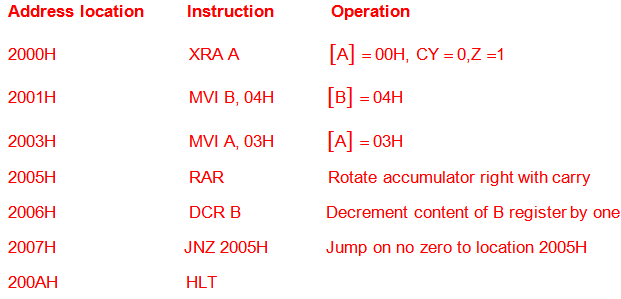Q6.
Consider the following Sum of Products expression, F.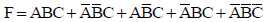The equivalent Product of Sums expression is
Explaination / Solution:Q7. In the following sequential circuit, the initial state (before the first clock pulse) of the circuit is Q1Q0 = 00. The state (Q1Q0), immediately after the 333rd clock pulse isExplaination / Solution: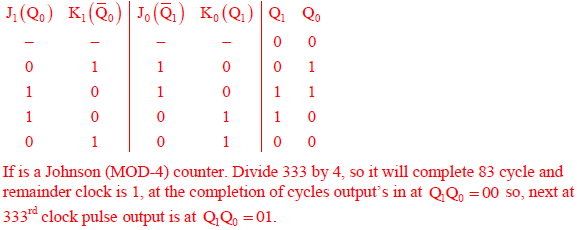Q8. A Boolean function f(A,B,C,D) = ∏(1,5,12,15) is to be implemented using an 8×1 multiplexer (A is MSB). The inputs ABC are connected to the select inputs S2S1S0 of the multiplexer respectively.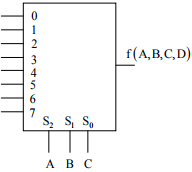Which one of the following options gives the correct inputs to pins 0,1,2,3,4,5,6,7 in order?
Explaination / Solution: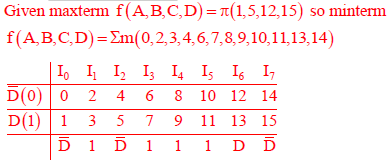Q9.
Consider the following circuit which uses a 2-to-1 multiplexer as shown in the figure below. The Boolean expression for output F in terms of A and B is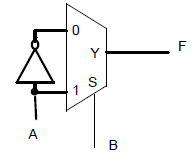Explaination / Solution:Q10. The current state QAQB of a two JK flip-flop system is 00. Assume that the clock rise-time is much smaller than the delay of the JK flip-flop. The next state of the system is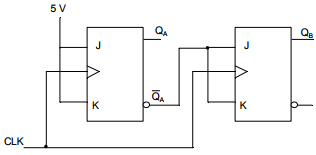Explaination / Solution: